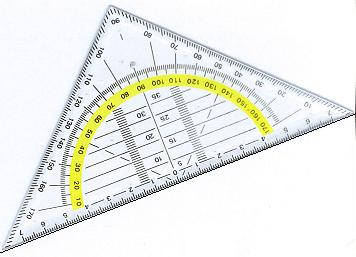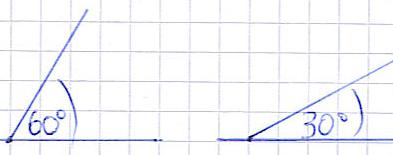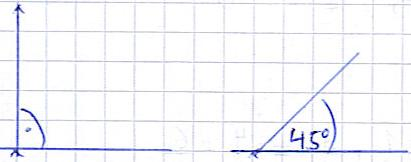# 1 degree is an acute angle

## Geometry: Set square and angles (degrees)

This article deals with the use of the set square and the entry of angles. Then you should know how to enter angles in a drawing and what different angle names there are.The set square is a drawing device for mathematics, which enables so-called angles to be entered. Now some will ask: what is an angle? Well, imagine two straight lines that intersect. An angle forms between these. We'll go into this with a few examples. Before that, however, first of all a graphic that shows you what a set square actually is:Now the question arises: What does an angle look like and how do I actually draw something like that? First of all, a few pictures showing the angle entries. The first example shows an angle of 60 degrees, the second example shows an angle of 30 degrees.And this is how it works if you have to enter an angle yourself:

• Creates the set square to the straight line, as was done for the line in the set square graphic from this article (the first graphic of the article).
• Make sure that the "0" is at the point where the two straight lines should intersect. There you make a small "x" to know where the intersection should be.
• At the top of the set square you can see the numbers 10, 20, 30 ... 170. If you want to draw an angle of 60 degrees, put an "x" in the place where it says 60 degrees (on the paper, not on the set square) .
• Connect the two xs with a line.
Show:

### 90 degrees and 45 degrees angles

The 90 degree angle and the 45 degree angle are two "important" angles in geometry. These occur again and again and are required for different applications. The following graphic shows you what these two angles look like.The first example shows a 90 degree angle. To mark this, a quarter circle is drawn between the straight lines and a point is set. The second example shows a 45 degree angle.

Show:

### Different types of angles

There are different types of angles in mathematics. Different names are given depending on the number of degrees:

• A 0 degree angle is a zero angle.
• 0 to 90 degrees is known as an acute angle.
• Exactly 90 degrees is a right angle.
• 90 to 180 is called an obtuse angle.
• A 180 degree angle is a straight angle.
• 180 to 360 degrees is an obtuse angle.
• A full angle is exactly 360 degrees.

### Working with the opposite angle, 360 degree angle

Take another look at the right angle. And imagine if the angle got even bigger. Then at some point 180 degrees will be reached, then 270 and if the two straight lines are on top of each other, you have reached a 360 degree angle. The stupid thing about the set square is that it only shows the degrees from 0 to 180. So what do you do if you want to draw 200 degrees or 260 degrees? There is a little trick to do this:

• Subtract 180 from the number of degrees you want to reach. So if you want to draw in 200 degrees, for example, calculate 200 degrees - 180 degrees = 20 degrees.
• Now rotates the set square itself by 180 degrees counterclockwise. The set square from the graphic above would therefore point with the tip down.
• Now enter the 20 degrees, as already explained in the previous section.

Left:

### Who's Online

We have 769 guests online